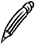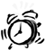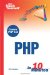Array Functions

You have already seen the array function used to generate an array from a list of values. Now let's take a look at some of the other functions PHP provides for manipulating arrays.

There are many more array functions in PHP than this book can cover. If you need to perform a complex array operation that you have not learned about, refer to the online documentation at www.php.net/ref.array.

Sorting

To sort the values in an array, you use the sort function or one of its derivatives, as in the following example:

sort(\$temps);Sorting Functions sort and other related functions take a single array argument and sort that array. The sorted array is not returned; the return value indicates success or failure.

Sorting the original \$temps array with sort arranges the values into numeric order, but the key values are also renumbered. After you perform the sort, index 0 of the array will contain the lowest value from the array, and there is no way of telling which value corresponds to each month.

You can use asort to sort an array while maintaining the key associations, whether it is an associative array or numerically indexed. After you sort \$temps, index 0 will still contain January's average temperature, but if you loop through the array, the elements will be retrieved in sorted order.

Using the associative array \$temps as an example, the following code displays the months and their average temperatures, from coldest to hottest:

\$temps = array("jan" => 38, "feb" => 40, "mar" => 49,                "apr" => 60, "may" => 70, "jun" => 79,                "jul" => 84, "aug" => 83, "sep" => 76,                "oct" => 65, "nov" => 54, "dec" => 42); asort(\$temps); foreach(\$temps as \$month => \$temp) {   print "\$month: \$temp <br>\n"; }

It is also possible to sort an array on the keys rather than on the element values, by using ksort. Using ksort on the associative \$temps array arranges the elements alphabetically on the month name keys. Therefore, when you loop through the sorted array, the first value fetched would be \$temps["apr"], followed by \$temps["aug"], and so on.

To reverse the sort order for any of these functions, you use rsort in place of sort. The reverse of asort is arsort, and the reverse of ksort is krsort. To reverse the order of an array as it stands without sorting, you simply use array_reverse.

Randomizing an Array

As well as sorting the values of an array into order, PHP provides functions so that you can easily randomize elements in an array.

The shuffle function works in a similar way to the sorting functions: It takes a single array argument and shuffles the elements in that array into a random order. As with sort, the key associations are lost, and the shuffled values will always be indexed numerically.

Set Functions

By treating an array as a set of values, you can perform set arithmetic by using PHP's array functions.

To combine the values from different arrays (a union operation), you use the array_merge function with two or more array arguments, as in the following example:

\$union = array_merge(\$array1, \$array2, \$array3, ...);

A new array is returned that contains all the elements from the listed arrays. In this example, the \$union array will contain all the elements in \$array1, followed by all the elements in \$array2, and so on.

To remove duplicate values from any array, you use array_unique so that if two different index keys refer to the same value, only one will be kept.

The array_intersect function performs an intersection on two arrays. The following example produces a new array, \$intersect, that contains all the elements from \$array1 that are also present in \$array2:

\$intersect = array_intersect(\$array1, \$array2);

To find the difference between two sets, you can use the array_diff function. The following example returns the array \$diff, which contains only elements from \$array1 that are not present in \$array2:

\$diff = array_diff(\$array1, \$array2);

Looking Inside Arrays

The count function returns the number of elements in an array. It takes a single array argument. For example, the following statement shows that there are 12 values in the \$temps array:

echo count(\$temps);

To find out whether a value exists within an array without having to write a loop to search through every value, you can use in_array or array_search. The first argument is the value to search for, and the second is the array to look inside:

if (in_array("PHP", \$languages)) {   ... }

The difference between these functions is the return value. If the value exists within the array, array_search returns the corresponding key, whereas in_array returns only a Boolean result.Needle in a Haystack Somewhat confusingly, the order of the needle and haystack arguments to in_array and array_search is opposite that of string functions, such as strpos and strstr.

To check whether a particular key exists in an array, you use array_key_exists. The following example determines whether the December value of \$temps has been set:

if (array_key_exists("dec", \$temps)) {   ... }

Serializing

The serialize function creates a textual representation of the data an array holds. This is a powerful feature that gives you the ability to easily write the contents of a PHP array to a database or file.

Lessons 17, "Filesystem Access," and 19, "Using a MySQL Database," deal with the specifics of filesystem and database storage. For now let's just take a look at how serialization of an array works.

Calling serialize with an array argument returns a string that represents the keys and values in that array, in a structured format. You can then decode that string by using the unserialize function to return the original array.

The serialized string that represents the associative array \$temps is as follows:

a:12:{s:3:"jan";i:38;s:3:"feb";i:40;s:3:"mar";i:49; s:3:"apr";i:60; s:3:"may";i:70;s:3:"jun"; i:79;s:3:"jul";i:84;s:3:"aug";i:83;s:3:"sep"; si:76;s:3:"oct";i:65;s:3:"nov";i:54;s:3:"dec";i:42;}

You can probably figure out how this string is structured, and the only argument you would ever pass to unserialize is the result of a serialize operationthere is no point in trying to construct it yourself.Sams Teach Yourself PHP in 10 Minutes
ISBN: 0672327627
EAN: 2147483647
Year: 2005
Pages: 151
Authors: Chris Newman

Similar book on Amazon